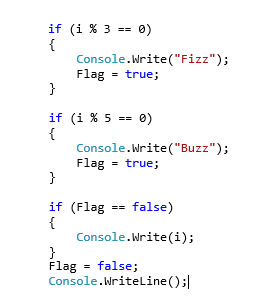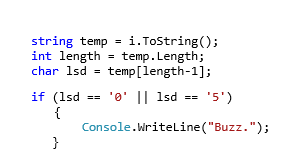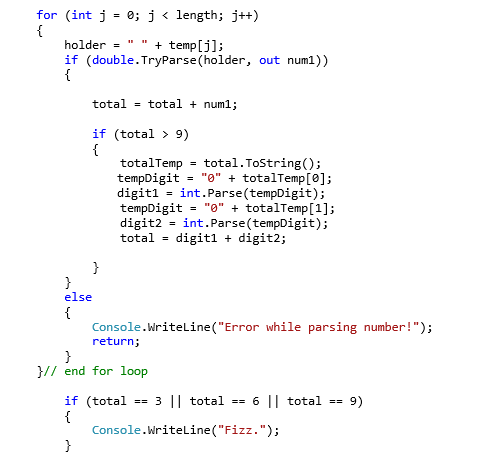## FizzBuzz Fun

My FizzBuzz Approach

FizzBuzz is one of those near legendary software interview questions that I’ve repeatedly been told of but never actually encountered during an interview, which sort of makes me sad.  So I decided to bat it around like a kitten would a ball of string and see what fun I could have coming up with less than obvious approaches to solving what’s meant to be a pretty simple problem. Here we go!

The Problem: FizzBuzz is a simple enough problem that asks the programmer to create an algorithm that takes numbers as input and produces output based on analysis of that input. Specifically, if the input is a number divisible by 3 the application should output ‘Fizz’, and if the input is divisible by 5 the output should read ‘Buzz’. In the event both cases are true the output should be ‘FizzBuzz’. All other cases should simple parrot the input as output.

The Approach: I think the most obvious solution is to just do exactly what the problem description asks, i.e. evaluate the input by dividing it by 3, by 5, and then by 3 and 5. A very easy way of determining if a number is divisible by another number is to use the mod operator and evaluate the remainder. So, something like this:I’m sort of cutting a corner here by never actually checking to see if both cases are true. Instead I’m cheating by not adding new lines after each print statement, so should both evaluate true Fizz will first print, followed by Buzz, which will appear together as FizzBuzz on the line.  The use of a flag allows for the function to know if it needs to print the input or not, depending on if Fizz or Buzz are found. It can definitely be argued this is against the spirit of the game, since it supposed originated as a children’s’ game for teaching division.

A slightly less cheaty approach, as suggested by my friend JameyC would be:In this case it’s much more obvious if both checks were passed simultaneously or not, and it’s probably more in the spirit of the game to begin with. In this approach FizzBuzz is evaluated before looking for Fizz or Buzz separately, so the combine case is already ruled out before either single case is even considered. Also, since only FizzBuzz has already failed if Fizz or Buzz are being evaluated on their own, we know that only one of the two can return true, allowing for a waterfall of nested if statements.

There’s a whole wealth of permutations of these approaches with different advantages and disadvantages, but I wanted to try something a little more fun. That’s what lead me to wonder about basically ignoring the actual meaning of the input values, and deconstructing them into forms that could be more directly evaluated by employing properties of 3 and 5. Specifically, the properties that mean any number divisible by 3 will yield a smaller value also divisible by 3 when its digits are summed, and the fact any number divisible by 5 will end with either a 0 or a 5.

First the easier of the approaches, as stated above, any multiple of 5 is only capable of having a 5 or 0 in the ones column because 5 is exactly half of 10, which resets the column. This means multiples of 5 will follow a repeating pattern of 5s and 0s. That’s very obvious, but I felt like I should make an attempt to explain it anyway for consistency sake. So keeping this in mind we can eliminate the second mod operator by figuring out if the final digit is a 0 or 5 with simple logic.

Since I was already employing String conversions for this attempt, I decided to continue the theme and just isolate the least significant digit of the input and check to see if it held 0 or 5.Nice and simple, though now with the overhead of dealing with strings. Now for the threes!

Dealing with threes means exploiting the property shared by all numbers divisible by three that I mentioned earlier. For example, 999 is divisible by 3, as are the results of 9+9+9=27 and then 2+7=9.  Every two digit or higher result can be broken down until finally a one digit number is found. At this point all that remains is checking to see if that single digit is equal to 3, 6, or 9, the only values smaller than 10 that are divisible by 3. This involves a lot of addition of course, but I liked the appeal of solving a division problem using only addition.In addition to being a fun, maybe less obvious approach to FizzBuzz, I like to think this approach is probably much closer to what children would do in their minds when playing the original game.

Of course things get messy here when it comes to breaking numbers into a series of single digits. This is a problem with about as many solutions as there are languages, because a great many approaches are based on the quirks of the language they’re implemented in. One of the more universal, though not particularly efficient, is the approach I used with converting the input into a string. From here I could treat numbers as simply arrays of digits, and convert them one by one back to ints by parsing each index one at a time.

Thirdly I wanted to toy around with an approach that put a recursive spin on things. This is admittedly a horrible, horrible option, and is almost guaranteed to cause a stack overflow for large input values, but in a quest for fun and novelty I feel it has its merits.

This is a very simple to code approach that uses the same algorithm for 3s and 5s with only the number being checked for and the size of the decrement step being changed. I’m back to using the trick from the original approach, so the two functions don’t print new line characters, enabling FizzBuzz to be printed without either function knowing what the other has found. The only communication between the two recursive functions and the calling function is the value of the Flag, which is declared in the base function and passed to both recursive functions by reference. When either recursive function prints to the console, the flag is set so the calling function knows to only print a newline character and not the input.Full code can be found here!